NCERT Solutions for Class 10 Maths Chapter 6 Triangles Ex 6.2 are part of NCERT Solutions for Class 10 Maths. Here we have given NCERT Solutions for Class 10 Maths Chapter 6 Triangles Ex 6.2.

 Board CBSE Textbook NCERT Class Class 10 Subject Maths Chapter Chapter 6 Chapter Name Triangles Exercise Ex 6.2 Number of Questions Solved 10 Category NCERT Solutions

## NCERT Solutions for Class 10 Maths Chapter 6 Triangles Ex 6.2

Question 1.
In the given figure (i) and (ii), DE || BC. Find EC in (i) and AD in (ii).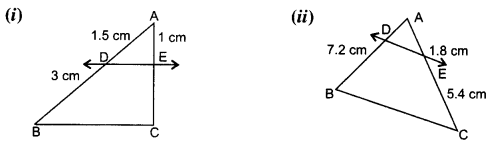Solution: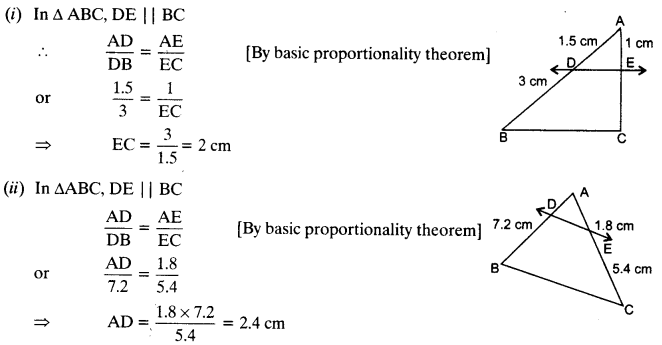Question 2.
E and F are points on the sides PQ and PR respectively of a ∆PQR. For each of the following cases, state whether EF || QR:
(i) PE = 3.9 cm, EQ = 3 cm, PF = 3.6 cm and FR = 2.4 cm
(ii) PE = 4 cm, QE = 4.5 cm, PF = 8 cm and RF = 9 cm
(iii) PQ = 1.28 cm, PR = 2.56 cm, PE = 0.18 cm and PF = 0.36 cm
Solution: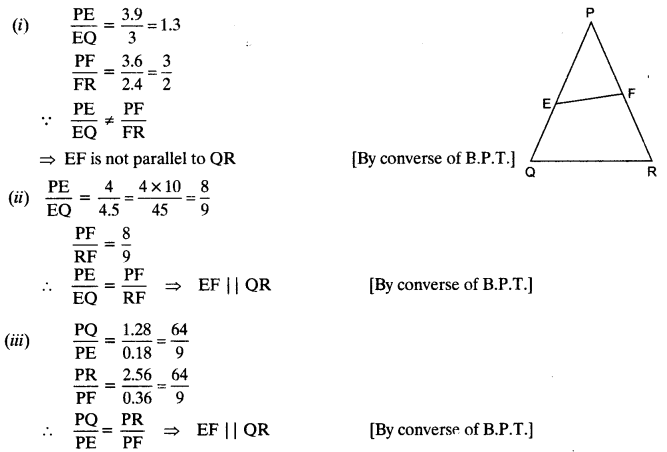Question 3.
In the given figure, if LM || CB and LN || CD.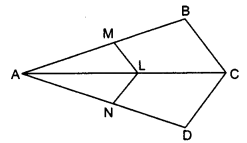Solution: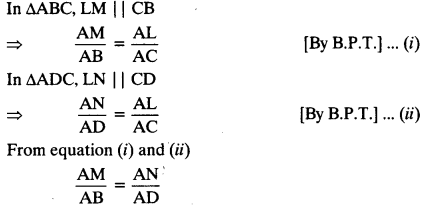Question 4.
In the given figure, DE || AC and DF || AE.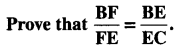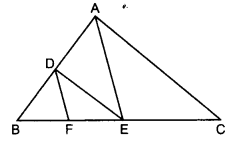Solution: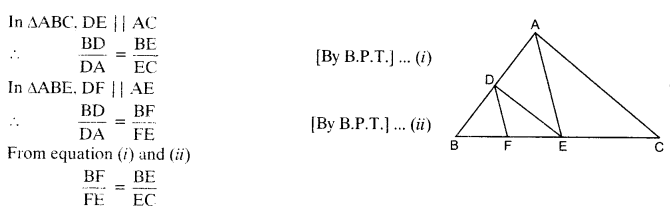Question 5.
In the given figure, DE || OQ and DF || OR. Show that EF || QR.Solution:Question 6.
In the given figure, A, B and C are points on OP, OQ and OR respectively such that AB || PQ and AC || PR. Show that BC || QR.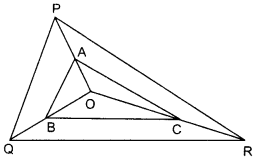Solution: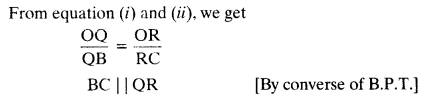Question 7.
Using B.P.T., prove that a line drawn through the mid-point of one side of a triangle parallel to another side bisects the third side.
Solution:
Given: A ∆ABC in which D is the mid-point of AB and DE || BCQuestion 8.
Using converse of B.P.T., prove that the line joining the mid-points of any two sides of a triangle is parallel to the third side.
Solution:
Given: A ΔABC in which D and E are mid-points of sides AB and AC respectively.
To Prove: DE || BC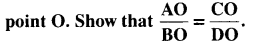Question 9.
ABCD is a trapezium in which AB || DC and its diagonals intersect each other at the
Solution: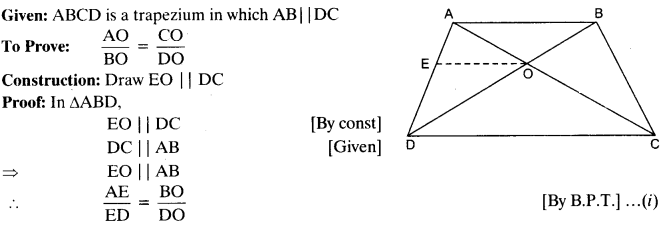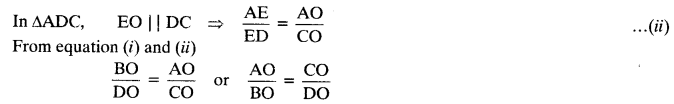Question 10.
The diagonals of a quadrilateral ABCD intersect each other at the point O such that $$\frac { AO }{ BO }$$ = $$\frac { CO }{ DO }$$ Show that ABCD is a trapezium.
Solution: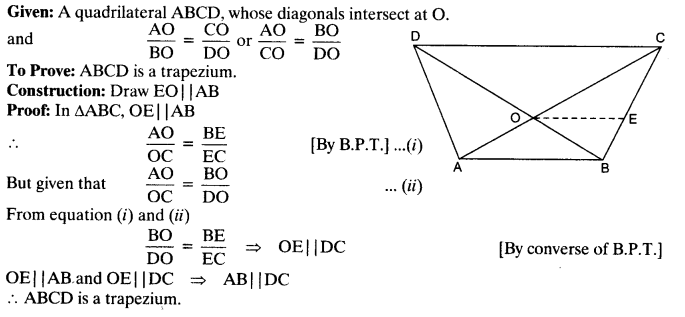We hope the NCERT Solutions for Class 10 Maths Chapter 6 Triangles Ex 6.2 help you. If you have any query regarding NCERT Solutions for Class 10 Maths Chapter 6 Triangles Ex 6.2, drop a comment below and we will get back to you at the earliest.